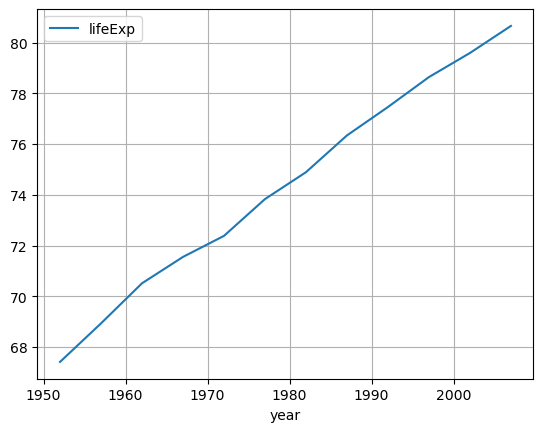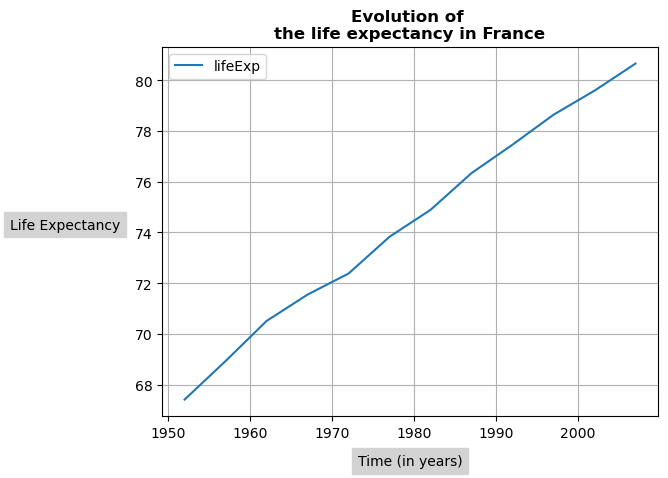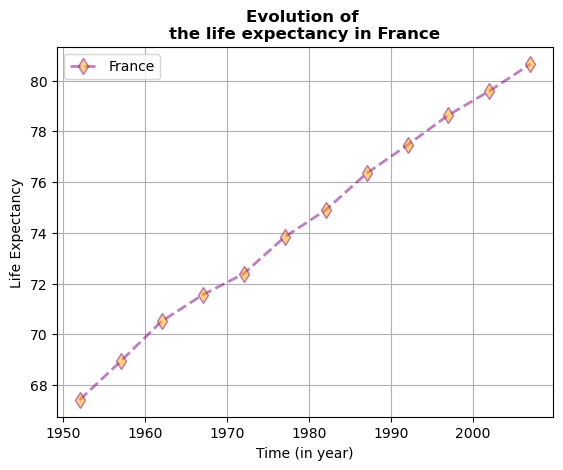A line chart is a graphical representation of the evolution of a variable over a continuous range, where data points are connected by lines to show the trend and variation in the data. Line charts display the data as a continuous line.
Pandas, a powerful data manipulation library in Python, allows us to create line charts easily. In this post, we will explore how to leverage `Pandas` to customize line charts, making it good looking and studying available options.

## Libraries

Pandas is a popular open-source Python library used for data manipulation and analysis. It provides data structures and functions that make working with structured data, such as tabular data (like `Excel` spreadsheets or `SQL` tables), easy and intuitive.

To install Pandas, you can use the following command in your command-line interface (such as `Terminal` or `Command Prompt`):

`pip install pandas`

Matplotlib functionalities have been integrated into the pandas library, facilitating their use with `dataframes` and `series`. For this reason, you might also need to import the matplotlib library when building charts with Pandas.

This also means that they use the same functions, and if you already know Matplotlib, you'll have no trouble learning plots with Pandas.

``````import pandas as pd
import matplotlib.pyplot as plt``````

## Dataset

In order to create graphics with Pandas, we need to use pandas objects: `Dataframes` and `Series`. A dataframe can be seen as an `Excel` table, and a series as a `column` in that table. This means that we must systematically convert our data into a format used by pandas.

Since histograms need quantitative variables, we will get the Gap Minder dataset using the `read_csv()` function. The data can be accessed using the url below.

To have just one line, we create a subset of our data frame to select only the rows for France.

``````url = 'https://raw.githubusercontent.com/holtzy/The-Python-Graph-Gallery/master/static/data/gapminderData.csv'

# Subset rows for France only
df_france = df[df['country']=='France']``````

## Basic line chart

Once we've opened our dataset, we'll now create the graph. The following displays the evolution of the life expectancy using the `plot()` function. Also, keep in mind that the `kind='line'` argument is facultative (you can remove it!) since it's the default value when calling the `plot()` function.

``````# Create and display the linechart
df_france.plot(x='year',
y='lifeExp',
kind='line', # (facultative) Default argument
grid=True, # Add a grid in the background
)
plt.show()``````## Custom axis and title

Adding titles and names to axes with Pandas requires a syntax very similar to that of matplotlib.

Here we use the `set_title()` and `set_xlabel()` (and `set_ylabel()`) functions to add them. We add the `weight='bold'` argument so that the title really looks like a title. I give you example of customizations but but you can change them the way you want!

``````ax = df_france.plot(x='year',
y='lifeExp',
grid=True)

# Add a bold title ('\n' allow us to jump rows)
ax.set_title('Evolution of \nthe life expectancy in France',
weight='bold')

ax.set_ylabel('Life Expectancy',
rotation=0, # Rotate it (default to 90)
backgroundcolor='lightgray' # Background color
)
ax.set_xlabel('Time (in years)',
backgroundcolor='lightgray' # Background color
)

# Show the plot
plt.show()``````## Change line style

The line is highly customizable:

• `color` argument for the color of the line
• `linewidth` argument for the width of the line
• `alpha` argument for the opacity of the line: between 0 (lowest) and 1 (highest)
• `label` argument for the text in the legend
• add markers at each point and change their style

Example of properties of the markers:

• shape with the `marker` argument (lots of possibilities here: `'.'`, `','`, `'o'`, `'v'`, `'^'`, `'<'`, `'>'`, `'s'`, `'p'`, `'*'`, `'+'`, `'x'`, `'D'`, `'d'`, `'h'`, `'H'`, `'1'`, `'2'`, `'3'`, `'4'`)
• size wither the `markersize` argument
• color with the `makerfacecolor` argument)
``````# Customize the line style, color, and width
ax = df_france.plot(x='year',
y='lifeExp',
grid=True,
linestyle='--',
alpha=0.5, # Opacity
color='purple',
linewidth=2.0, # Width
marker='d',  # Markers shape
markersize=8,  # Markers size
markerfacecolor='orange', # Markers color
label='France'
)

# Add a bold title ('\n' allow us to jump rows)
ax.set_title('Evolution of \nthe life expectancy in France',
weight='bold')

ax.set_ylabel('Life Expectancy')
ax.set_xlabel('Time (in year)')

# Show the plot
plt.show()``````## Going further

This post explains how to customize a line chart built with pandas.

For more examples of how to create or customize your line charts, see the line charts section. You may also be interested in how to created an area chart.

## Contact & Edit

👋 This document is a work by Yan Holtz. You can contribute on github, send me a feedback on twitter or subscribe to the newsletter to know when new examples are published! 🔥